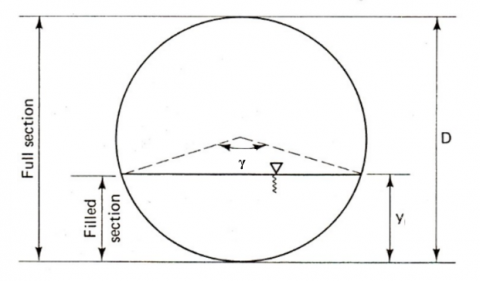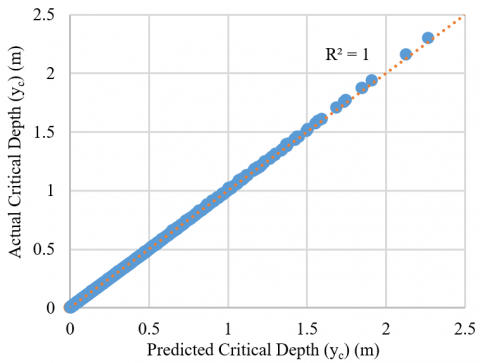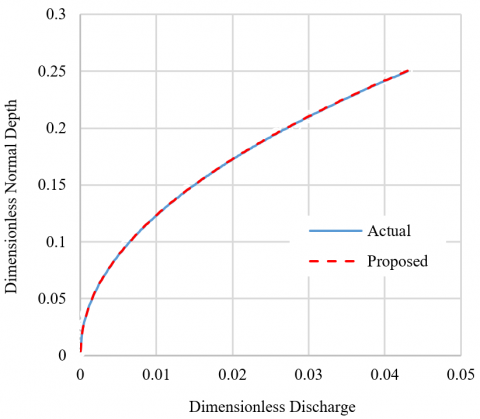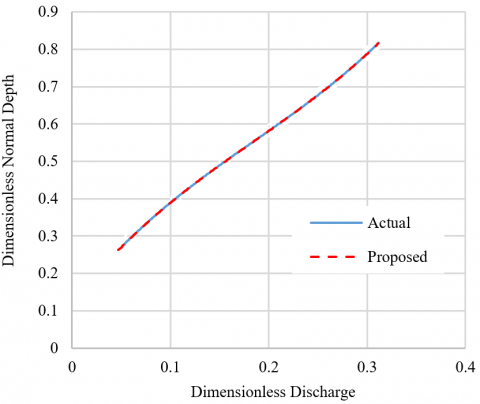# Novel Approach to Computing Critical and Normal Depth in Circular Channels

Novel Approach to Computing Critical and Normal Depth in Circular Channels

Akram K. Mohammed Raad H. Irzooki Asmaa A. Jamel Wesam S. Mohammed-Ali* Suhad S. Abbas

Dept. of Environmental Engineering, College of Engineering, Tikrit University, Tikrit 34001, Iraq

Dept. of Civil Engineering, College of Engineering, Tikrit University, Tikrit 34001, Iraq

Dept. of Civil Engineering, University of Technology, Baghdad 10011, Iraq

Corresponding Author Email:
wsm27f@mst.edu
Page:
923-927
|
DOI:
https://doi.org/10.18280/mmep.080611
14 August 2021
|
Accepted:
10 October 2021
|
Published:
22 December 2021
| Citation

OPEN ACCESS

Abstract:

The critical depth and normal depth computation are essential for hydraulic engineers to understanding the characteristics of varied flow in open channels. These depths are fundamental to analyze the flow for irrigation, drainage, and sewer pipes. Several explicit solutions to calculate critical and normal depths in different shape open channels were discovered over time. Regardless of the complexity of using these explicit solutions, these formulas have a significant error percentage compared to the exact solution. Therefore, this research explicitly calculates the normal and critical depth in circular channels and finds simple, fast, and accurate equations. First, the dimensional analysis was used to propose an analytical equation for measuring the circular channels' critical and normal depths. Then, regression analysis has been carried for 2160 sets of discharge versus critical and normal depths data in a circular open channel. The results show that this study's proposed equation for measuring the circular channels' critical and normal depths overcomes the error percentage in previous studies. Furthermore, the proposed equation offers efficiency and precision compared with other previous solutions.

Keywords:

critical depth, normal depth, circular channel, dimensional analysis, statistical analysis

1. Introduction

The depth of flow associated with a uniform flow is called the normal depth. On the other hand, the flow depth corresponding to the minimum specific energy for a given discharge in a waterway is called a critical depth. The critical depth and normal depth computation are crucial for hydraulic engineers to analyze varied flow in open channels for irrigation, drainage, and sewer pipes [1, 2]. These depths are essential to understanding the flow characteristics. The normal depth in open channels can be defined as the depth of flow that occurs when the flow is steady and uniform. While critical depth is established when the specific energy is at a minimum value for a certain discharge. When the normal depth is higher than the critical depth, then the flow is classified as a subcritical flow, but the flow becomes supercritical flow when the normal depth is less than the critical depth.

There is only one normal depth for a given situation of channel geometry and discharge. The normal depth is typically calculated using Manning's Equation, which requires an iterative solution to compute this depth in circular channels. Simultaneously, many methods were utilized for computing the critical depth, for instance, the Algebraic method, Graphical method, Design chart, Numerical method, Bi-section method, Newton Raphson method, and Semi empirical approach .

Due to governing equations' implicit nature, a direct solution for normal and critical depths is not feasible [4, 5]. Thus, the researchers proposed developing a computer program to avoid repetitive computations. Trial procedures and graphical methods are currently used to determine the critical depth [6-8]. An explicit solution for critical depth and normal depths in circular and different shape channels was established based on regression equations that improved the equations' accuracy and increased their complexity . Recently, three explicit solutions for critical depth in circular, arched, and egg-shaped sections are driven . Other researchers focused in their studies on finding an explicit solution for calculating the critical depth and normal depths of non-circular section open channels, such as trapezoidal, parabolic, ovoidal, and horseshoe shapes [11, 12]. These formula forms are simple, and the physical concept was obvious. However, these formulas have a significant percentage of error compared to the exact solution. On the same level, other formulas presented accurate results, but they require much effort and time to calculate the critical depth [7, 13, 14].

An investigation of the literature confirmed that a direct solution for normal and critical depths has a significant development in recent times. However, none of the reviewed studies addressed this work's core motivations. Therefore, this research is presented herein to explicitly calculate the normal and critical depth in circular channels to address this gap and find simple, fast, and accurate equations.

2. Flow Formula for Circular Channel

Considering Figure 1 for a partially filled circular channel section, the discharge Q carried by this channel using Manning's Equation is:

$Q=\frac{1}{n} A R^{\frac{2}{3}} S^{\frac{1}{2}}$       (1)

$A=\frac{D^{2}}{8}\left(\gamma-\sin ^{2} \gamma\right)$       (2)

$P=\frac{\gamma}{2} . D$       (3)

## 1.pngFigure 1. Partially flow in a circular open channel

$R=\frac{A}{P}=\frac{\gamma D\left(\gamma-\sin ^{2} \gamma\right)}{16}$       (4)

$T=D \sin ^{2} \frac{\gamma}{2}$       (5)

$\gamma=2 \cos ^{-1}(1-2 \eta)$       (6)

$Q=\frac{D^{2}}{8 n}\left(\gamma-\sin ^{2} \gamma\right)\left(\frac{\gamma D\left(\gamma-\sin ^{2} \gamma\right)}{16}\right)^{\frac{2}{3}} S^{\frac{1}{2}}$       (7)

where, D is channel diameter, γ is water surface angle in radians (which depends on the flow depth yn), n is Manning's roughness coefficient, S is the bed slope of channel, and h=yn/D.

Eq. (7) is an implicit equation, as the depth of flow can be found by the trial-and-error method.

The critical flow condition in a circular open channel is described by the following relationship .

$\frac{\alpha Q^{2}}{g} \cdot \frac{T_{C}}{A_{C}^{3}}=1$       (8)

where, α is the energy correction factor and g is the acceleration due to gravity.

Substituting for A and T from Eqns. (2) and (5), for critical condition, into Eq. (8) yields the following dimensionless form:

$\frac{\alpha Q^{2}}{g}=\frac{A_{C}^{3}}{T_{C}}$

$\frac{\alpha Q^{2}}{g}=\frac{\left(\frac{D^{2}}{8}\left(\gamma_{c}-\sin ^{2} \gamma_{c}\right)\right)^{3}}{D \sin \frac{\gamma c}{2}}=\frac{D^{5}\left(\gamma_{c}-\sin ^{2} \gamma_{c}\right)^{3}}{512 \sin \frac{\gamma c}{2}}$

$\frac{\alpha Q^{2}}{g D^{5}}=\frac{\left(\frac{\gamma_{c} \pi}{180}-\sin ^{2} \gamma_{c}\right)^{3}}{512\left(\sin \frac{\gamma c}{2}\right)}$

$\varepsilon=\frac{\alpha Q^{2}}{g D^{5}}$       (9)

$\varepsilon=\frac{\left(\frac{\gamma_{c} \pi}{180}-\sin \gamma_{c}\right)^{3}}{512\left(\sin \frac{\gamma c}{2}\right)}$       (10)

For calculating the critical depth in the circular channel, Eq. (10) must be solved. Eq. (10) is also implicit and can be solved by the trial-and-error method. Many researchers have suggested several explicit solutions for solving this problem.

In this paper, specific and explicit equations were derived for finding the critical and normal depths of the circular channels by statistical analysis depending on the dimensional analysis theory and curve fitting method, respectively. The methodology starts by computing the channel's discharge for many different geometries. Six different diameters of the circular channel D were used (0.5, 1, 1.5, 2, 2.5, and 3m). For each diameter, five different values of Manning's n (0.01, 0.015, 0.02, 0.025 and 0.03), four longitudinal bed slopes of the channel S (0.01, 0.001, 0.0001 and 0.00005), and eighteen normal depths yn were used. For each case, the discharge Q was calculated using Manning's Equation (Eq. (7)), and then the parameter ε and critical depth yc was calculated by trial-and-error method. The number of generated data of discharge versus critical and normal depth reached 2160 cases.

3. Dimensional Analysis

Dimensional analysis is a widely reliable tool for recognizing physical quantity relationships' knowledge structure in a detailed and consistent manner. It all begins because descriptive quantities in quantitative natural science have dimensions and can be divided into fundamental and derived quantities accordingly [15-19]. In this article, dimensional analysis was used to propose an analytical equation for measuring the circular channels' critical and normal depths.

3.1 Critical depth

Eq. (10) shows that the critical depth yc in the circular channel depended on the following variables:

Q=Discharge of flow (L3T-1).

D=Diameter of conduit (L).

g=Acceleration due to gravity (LT-2).

Therefore:

$y_{c}=f(Q, D, g)$        (11)

By applying the Π theorem, the following dimensionless parameters produce:

$\frac{y_{c}}{D}=\varphi_{1}\left(\frac{Q^{2}}{g D^{5}}\right)$        (12)

$\eta_{c c}=\varphi_{1}\left(\varepsilon_{c}\right)$        (13)

3.2 Normal depth

As shown in Eq. (7), the possible variables affecting the normal depth of flow yn through the circular channel are:

Q=Discharge of flow (L3T-1).

D=Diameter of conduit (L).

n=Manning's roughness coefficient (L-1/3T).

S=Conduit longitudinal slope.

Therefore:

$y_{n}=f(Q, D, n, S)$        (14)

The following dimensionless terms can be derived from the above Equation using the Π theorem:

$\frac{y_{n}}{D}=\varphi_{2}\left(\frac{n Q}{D^{\frac{8}{3}} S^{\frac{1}{2}}}\right)$        (15)

$\eta_{n c}=\varphi_{2}\left(\beta_{c}\right)$        (16)

4. Statistical Analysis Results

A statistical analysis and curve fitting were conducted as part of this study. Non-linear regression of the generated data was conducted using the IBM software SPSS Statistics to define an empirical model equation in line with the functional equation obtained by dimensional analysis.

4.1 Critical depth

The critical depth of flow across a circular channel was proposed using statistical analysis. The dimensionless parameters (ηcc, εc) of Eq. (10), which resulted from generated actual data, were first ordered in descending order, and then about two-thirds of them were included in the SPSS program.

The proposed Equation for critical depth is:

$\eta_{c c}=1.0115 \varepsilon_{c}^{0.254}$        (17)

Figure 2 shows the comparison between the remaining one-third generated critical depth data to the calculated critical depth from the above-proposed Equation. The coefficient of determination R2 of this relationship is equal to 1.

The maximum prospective error of Eq. (17) does not exceed 0.253% in the practical range (0.003≤hcc≤0.87).

## 2.pngFigure 2. Relation between the actual and predicted critical depth of a circular open channel

Also, an empirical formula was developed, Eq. (18), to compute the critical depth in a circular open channel that depends on the conduit's geometrical data.

$\eta_{c c}=0.8 \eta_{n c}^{0.999} \rho_{n c}^{0.257}$        (18)

where,

$\rho_{n c}=\left(\frac{S D^{\frac{1}{3}}}{g n^{2}}\right)$        (19)

Figure 3 shows the relation between the measured critical depth and that predicted from Eq. (18). The coefficient of determination R2 of this relationship is equal to 1.

## 3.pngFigure 3. Relation between the actual and predicted critical depth of a circular open channel

Figure 4 shows the dimensionless critical depth from the proposed equation and the actual critical depth through a circular channel. It can be seen a remarkable match between the expected and actual results.

## 4.pngFigure 4. Comparison between proposed and actual dimensionless critical depth

Table 1 listed the proposed and existing explicit equations for determining the critical depth in circular cross-section channels. Obviously, this paper's proposed equation offers efficiency and precision compared with other previous solutions.

Table 1. Proposed explicit solutions of critical depth through circular channels

 References Equation Application Rang Max. Relative Error % Straub  $\eta_{c c}=1.01 D^{-0.01} \varepsilon_{c}^{0.25}$ $0.1 \leq \eta_{c c} \leq 0.85$ 5.8 Swamee and Rathie  $\eta_{c c}=\left(1+0.77 \varepsilon_{c}^{-3}\right)^{-0.085}$ $0.02 \leq \eta_{c c} \leq 1$ 1.46 Vatankha and Bijankhan  $\eta_{c c}=\frac{0.9584 \varepsilon_{c}^{0.25}}{\left(1+0.106 \varepsilon_{c}^{0.26}-0.0132 \varepsilon_{c}^{1.863}\right)^{-10.022}}$ $0 \leq \eta_{c c} \leq 0.92$ 0.25 Vatankha and Easa  $\eta_{c c}=\left(1+3.83 \varepsilon_{c}^{-2.1454}-3.2 \varepsilon_{c}^{-2.1}\right)^{-0.115}$ $0.01 \leq \eta_{c c} \leq 1$ 0.27 Shang et al.  Proposed Eq. $\begin{gathered} \eta_{c c}=\left(1+3.83 \varepsilon_{c}^{-2.1454}-3.2 \varepsilon_{c}^{-2.1}\right)^{-0.115} \\ \eta_{c c}=1.0115 \varepsilon_{c}^{0.254} \end{gathered}$ $0.003 \leq \eta_{c c} \leq 0.87$ 0.25

Table 2. Proposed explicit solutions of normal depth through circular channels

 References Equation Application Rang Max. Relative Error % Vatankha and Easa  $\eta_{n c}=1.025 \beta_{c}^{\left(-0.55 \beta_{c}^{1.1}-14.55 \beta_{c}^{4.136}+0.4645\right)}$ $0.005 \leq \eta_{n c} \leq 0.82$ 0.35 Proposed Eq. $\eta_{n c}=1.1506 \beta^{0.4851}$ $0.003 \leq \eta_{c c} \leq 0.25$ 0 $\eta_{n c}=15.088 \beta_{c}^{3}-8.3569 \beta_{c}^{2}+3.3748 \beta_{c}+0.1202$ $0.25<\eta_{n_{c}} \leq 0.82$ 0

4.2 Normal depth

As mentioned above, the curve fitting method was utilized to establish an explicit equation for computing a normal depth of flow through circular conduits. Two equations are offered herein for calculating the normal depth in the circular channels depending on the value of the dimensionless normal depth $\eta_{c}$. The first equation is applied within limits of $\eta_{c}$ ranging from 0.003 0 to 0.25 or dimensionless discharge βc less than 0.043. The second equation applies to values of $\eta_{c}$ more than 0.25 to 0.82 or βc equal or more than 0.043. These two equations are:

$\eta_{n c}=1.1539 \beta_{c}^{0.4854}$        (20)

where, 0.003≤$\eta_{c}$≤0.25 or βc<0.043.

$\eta_{n c .}=14.995 \beta_{c}^{3}-3.3199 \beta_{r}^{2}+3.3722 \beta_{c}$        (21)

where, 0.25<βc≤0.82 or βc≥0.043.

The above two equations demonstrate the simplicity and fast at which they can find the normal depth. Their error ratio is approximately equal to zero when the results are compared to the actual depths. Table 2 shows the maximum relative error of the established equations compared to previous studies .

## 5.pngFigure 5. Comparison between proposed and actual dimensionless normal depth through a circular channel (0.003≤ηnc≤0.25)

## 6.pngFigure 6. Comparison between proposed and actual dimensionless normal depth through a circular channel (0.25< $\eta_{n_{c}}$ ≤0.82)

Furthermore, the results from Eqns. (20) and (21) in comparison to actual data are illustrated in Figure 5 and Figure 6, respectively.

5. Conclusions

An extensive dataset for discharge versus critical and normal depths was generated in this study. Then, specific and explicit equations were derived for finding the critical and normal depths of the circular channels by statistical analysis depending on the dimensional analysis theory and curve fitting method, respectively. Clearly, the proposed equation offers the simplest, practical, and accurate results compared with other earlier solutions.

While the proposed equation's assessment for measuring the critical and normal depth in circular channels was examined in this work, further investigation needs to be carried with sets of experimental datasets to provide must needed insight for the validity of this proposed equation.

References

 Raikar, R.V., Reddy, M.S., Vishwanadh, G.K. (2010). Normal and critical depth computations for egg-shaped conduit sections. Flow Measurement and Instrumentation, 21(3): 367-372. https://doi.org/10.1016/j.flowmeasinst.2010.04.007

 Subramanya, K. (2009). Flow in Open Channels. Tata McGraw-Hill Education.

 Swamee, P.K. (1993). Critical depth equations for irrigation canals. Journal of Irrigation and Drainage Engineering, 119(2): 400-409. https://doi.org/10.1061/(ASCE)0733-9437(1993)119:2(400)

 Akan, A.O. (2011). Open Channel Hydraulics. Butterworth-Heinemann.

 Moglen, G.E. (2015). Fundamentals of Open Channel Flow. CRC Press.

 Mohammed-Ali, W., Mendoza, C., Holmes Jr, R.R. (2020). Influence of hydropower outflow characteristics on riverbank stability: Case of the lower Osage River (Missouri, USA). Hydrological Sciences Journal, 65(10): 1784-1793. https://doi.org/10.1080/02626667.2020.1772974

 Straub, W.O. (1978). Quick and easy way to calculate critical and conjugate depths in circular open channels. Civil Engineering, 48(12), 70-71.

 Swamee, P.K., Rathie, P.N. (2005). Exact equations for critical depth in a trapezoidal canal. Journal of Irrigation and Drainage Engineering, 131(5): 474-476. https://doi.org/10.1061/(ASCE)0733-9437(2005)131:5(474)

 Vatankhah, A.R., Easa, S.M. (2011). Explicit solutions for critical and normal depths in channels with different shapes. Flow Measurement and Instrumentation, 22(1): 43-49. https://doi.org/10.1016/j.flowmeasinst.2010.12.003

 Shang, H., Xu, S., Zhang, K., Zhao, L. (2019). Explicit solution for critical depth in closed conduits flowing partly full. Water, 11(10): 2124. https://doi.org/10.3390/w11102124

 Arvanaghi, H., Mahtabi, G., Rashidi, M. (2015). New solutions for estimation of critical depth in trapezoidal cross section channel. J. Mater. Environ. Sci, 6(9): 2453-2460.

 Wang, Z. (1998). Formula for calculating critical depth of trapezoidal open channel. Journal of Hydraulic Engineering, 124(1): 90-91. https://doi.org/10.1061/(ASCE)0733-9429(1998)124:1(90)

 Vatankhah, A.R., Bijankhan, M. (2010). Choke-free flow in circular and ovoidal channels. In Proceedings of the Institution of Civil Engineers-Water Management, 163(4): 207-215. https://doi.org/10.1680/wama.2010.163.4.207

 Babaeyan-Koopaei, K. (2001). Dimensionless curves for normal-depth calculations in canal sections. Journal of Irrigation and Drainage Engineering, 127(6): 386-389. https://doi.org/10.1061/(ASCE)0733-9437(2001)127:6(386)

 Mohammed-Ali, W.S. (2012). Hydraulic characteristics of semi-elliptical sharp crested weirs. J. Int. J. v. Giraldez Rev. Civil Eng, 1: 13.

 Irzooki, R.H., Akib, S.M., Fayyadh, M.M. (2014). Experimental study of characteristics of flow over weirs with semicircular openings. Arabian Journal for Science and Engineering, 39(11): 7599-7608. https://doi.org/10.1007/s13369-014-1360-8

 Mohammed-Ali, W.S. (2011). The effect of middle sheet pile on the uplift pressure under hydraulic structures. European Journal of Scientific Research, 65(3): 350-359.

 Durst, F. (2008). Fluid mechanics: an introduction to the theory of fluid flows. Springer Science & Business Media.

 Khalaf, R.M., Irzooki, R.H., Shareef, S.J. (2014). Flow characteristics and energy dissipation over traditional and stepped spillway with semicircular crest. International Journal of Civil & Environmental Engineering IJCEE-IJENS, 14(2): 13-27.